# Electronics and Communication Engineering - Networks Analysis and Synthesis

1.

Three identical coils each having self inductance L are connected in series so that the fluxes of all the three are in the same direction. If mutual inductance between each pair of coils is M, the total inductance of the arrangement is

 A. 3 L + 9 M B. 1.5 L + 3 M C. 3 L + 3 M D. 3 L + 6 M

Explanation:

Total inductance = L + L + L + M + M + M + M + M + M = 3L + 6M.

2.

In a resistive ac circuit, the current __________ the voltage by __________ .

 A. lags, 90° B. lags, 45° C. lags, 0° D. leads 90°

Explanation:

In a resistive circuit the current is in phase with voltage, i.e., lags by 0°.

3.

KVL for second loop in figure is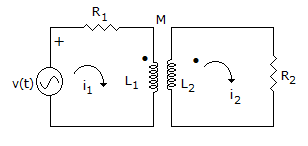A.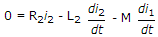B.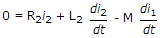C.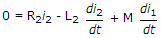D.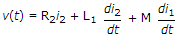Explanation:

emf induced in loop 2 due to i1 is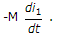4.

When two coupled coils of equal self inductance are cormected in series in one way the net inductance is 12 mH and when they are connected in the other way the net inductance is 4 mH. The maximum value of inductance when they are connected in parallel is

 A. 2 mH B. 3 mH C. 4 mH D. 6 mH

Explanation: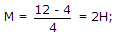L1 = L2 = L = 4H. When in parallel effective inductance =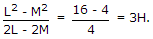5.

An R-L series circuit is excited by a voltage v(t). The circuit was initially relaxed. Which of the following sources of v(t) would cause maximum current at t = 0+?

 A. unit step B. unit impulse C. unit ramp D. unit step plus unit ramp